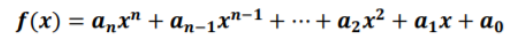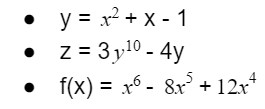# Polynomial Functions

 Would You Rather Listen to the Lesson?In precalculus, students learn about functions. In lessons on polynomial functions, students first learn how to define polynomial functions and how to find the zeros as well as roots of polynomial functions.

Even though precalculus lessons are often perceived as daunting for many students, they can be turned into a fun and easy learning experience given the right teaching strategies. To this end, we’ve prepared a few cool teaching strategies in this article.## Strategies to Teach Polynomial Functions

### What Are Polynomial Functions?

You can start your lesson by providing a definition of what polynomial functions are. You can define them as functions where f(x) is a polynomial in x. Point out that the general form of polynomial functions is like this:Add that in this general form, n represents a whole number. Highlight that in order to qualify as polynomial functions, these functions must be continuous, without breaks, holes, gaps, or sharp corners when graphed.

Write a few examples of polynomial functions on the whiteboard, such as:#### Degree of a Term

Once you have defined them, you can explain some of the terminology around polynomial functions. Explain to students that the degree of a term is the sum of the exponents of all the variables in that term. If the function has a single variable, the degree of a term is the exponent.

Provide a few examples:#### Leading Term in a Polynomial

Explain to students that the leading term in a polynomial is the term with the highest degree. Write a polynomial on the whiteboard, and illustrate to students how to identify the leading term in each of them:

For instance, in 2x³+ 6x²+5x- 1, the leading term is 2x³, because this term has the highest degree, that is, 3.

#### Leading Coefficient of a Polynomial

Explain to students that we refer to the coefficient of the leading term in a polynomial as the leading coefficient of this polynomial. Write an example of a polynomial and show students how to identify the leading coefficient.

For instance, in 2x³+ 6x²+5x- 1, we know that the leading term is 2x³. From here, we simply need to identify the coefficient in this term, which is 2. So the leading coefficient of this polynomial is 2.#### Constant Term in a Polynomial

Point out that the constant term in a polynomial is the term with degree 0. In other words, this is the term where there is no variable. Provide an example of how to identify the constant term in a polynomial.

For instance, write 2x³+ 6x²+5x- 1 on the whiteboard. To identify the constant term, we’ll look for the term where the variable doesn’t appear. This is – 1. So the constant term of 2x³+ 6x²+5x- 1 is – 1.

#### Degree of a Polynomial

You can define the degree of a polynomial as the degree of the leading term of the polynomial. Show students how to identify the degree of a given polynomial.

For example, in 2x³+ 6x²+5x- 1, we’ll first identify the leading term. As mentioned above, this is the term with the highest degree, i.e. 2x³. The degree of this term is 3. So the degree of the polynomial 2x³+ 6x²+5x- 1 is 3.

#### Roots/Zeros of a Polynomial Function

Explain that when we find the solution to a polynomial function, we’re finding the roots of that function. Underline that the roots are the same thing as the zeros of a polynomial function, that is, they’re the x-values that make the polynomial equal to zero.

In other words, P(x) = 0 for all zeros/roots of P(x).

### Launch a Video Lesson!

After having introduced the most important terms and definitions regarding polynomial functions, why not make your lesson more interesting by complementing it with a video? Moreover, a video lesson will greatly facilitate illustrating the different shapes of graphs.

This video is a great resource to use to present your lesson. The video contains a short introduction to polynomial functions, followed by instructions on how to find the zeros and roots of a given polynomial function. In addition, this video focuses on finding the zeros of polynomials.

## Activities to Practice Polynomial Functions

### Online Game

In this online game by Khan Academy, students will have the opportunity to practice finding the zeros (which is the same as roots) of polynomials. To use this activity in your classroom, make sure there are enough technical devices (one per student).

Explain to students that this is an individual activity and provide instructions. Students are presented with different polynomials where they’re asked to find the zeros and then plot them on the interactive graph. If they get stuck, they can choose to use a hint or watch a video for help.

## Before You Leave…

If you enjoyed these math strategies for teaching polynomial functions, you may want to check out our lesson that’s dedicated to this topic. So if you need guidance to structure your class and teach pre-calculus, make sure to sign up for more free resources here!

You can also sign up for our membership MathTeacherCoach or head over to our blog – you’ll find plenty of awesome resources for kids of all ages!